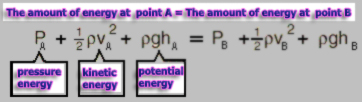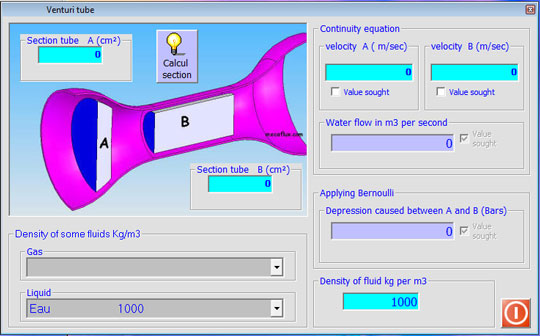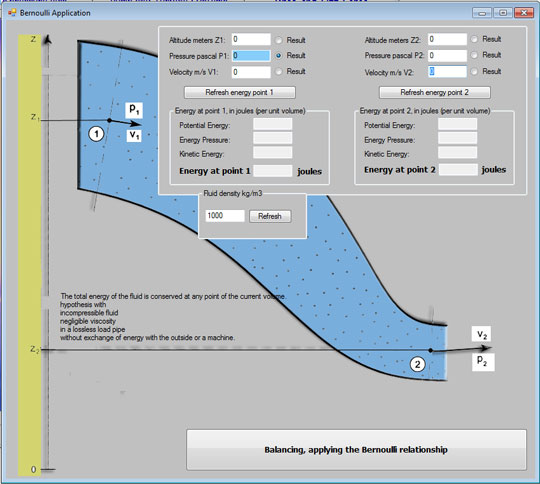according to the Bernoulli's Theorem

The Bernoulli equation can be considered as a principle of conservation of energy, suitable for moving fluids.The behavior usually called "Venturi effect" or "Bernoulli effect" is the reduction of fluid pressure in areas where the flow velocity is increased. This decrease in pressure in a narrowing of the duct may seem contradictory, unless you consider the pressure as an energy density. Passage through the narrowing, the fluid velocity, so its kinetic energy should be increased at the expense of pressure energy.Assuming that the flow is laminar, the major pressure losses of the duct are negligible, and that the speed is the average speed:

The calculation interface Venturi tube in tools MECAFLUX

This interface is used to calculate a venturi according section, density, flow or pressure measured. Whether for producing a flow meter, a vacuum system ...You can set in the "venturi" software tool mecaflux (Tools menu), The parameter desired by checking "sought value". This allows to find a fluid velocity (and thus a flow rate) according to a negative pressure caused, or to determine the depression generated by a certain speed or fluid flow through a cross-sectional constriction.

The tool "Bernoulli" of software Mecaflux (tools menu) allows the same type of calculation, but with the ability to define a height difference. Voir Bernoulli